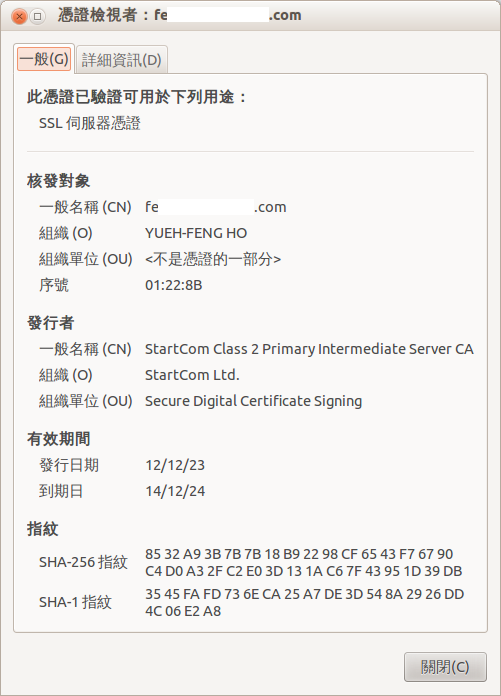# 公錀加密在開放式 Http 連線的應用```import os, base64, random
from Crypto.Cipher import AES
from Crypto.PublicKey import RSA
class AESCrypto:
u""" plain 文本的字串長度須為 16 的倍數
"""
BLOCK_SIZE = 32
return s + (self.BLOCK_SIZE - len(s) % self.BLOCK_SIZE) * self.PADDING
def EncodeAES(self, s):
def DecodeAES(self, e):
def __init__(self, secret=''):
if secret:
self.secret = secret
else:
self.secret = os.urandom(self.BLOCK_SIZE)
self.cipher = AES.new(self.secret)
def public_key_encypt_secret(self, public_key):
key = RSA.importKey(public_key)
return key.encrypt(base64.b64encode(self.secret),
random.randint(-10240000, 10240000))
if __name__ == '__main__':
ac = AESCrypto()
encoded = ac.EncodeAES('This is a TTTest')
decoded = ac.DecodeAES(encoded)
print decoded # will show up "This is a TTTest"
# 如果 some_file 是透過網路傳輸得來，通常它會是 b64encode 。
encypt_secret = ac.public_key_encypt_secret(public_key)
# encypt_secret 要回傳給私錀擁有者
print encypt_secret
```

```#!/usr/bin/env python
# -*- coding: utf8 -*-
import base64, random, urllib, json
from Crypto.PublicKey import RSA

def some_view_function(R):
key = RSA.generate(1024) #隨機生成公私錀
public_key = base64.b64encode(key.publickey().exportKey())
url = ('http://some.domain/some_where_funciton/?public_key=%s' % public_key)
fd = urllib.urlopen(url)
if str(fd.code) != '200': return HttpResponse('There are Some Error in "%s"'%url)